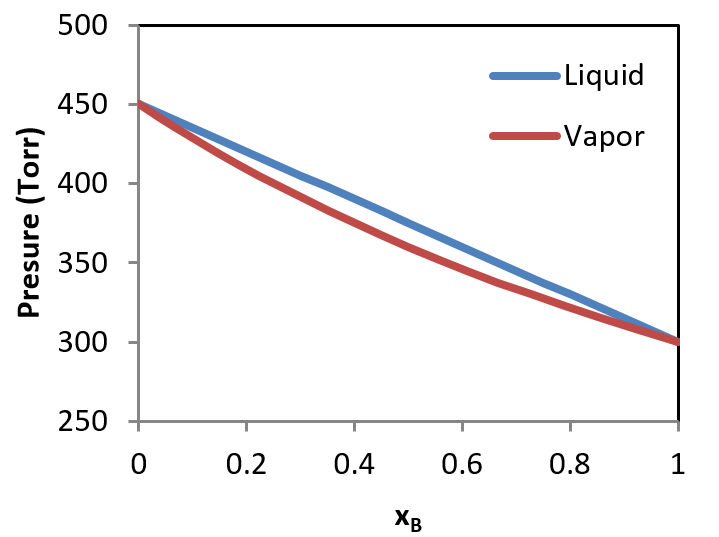# 8.7: Liquid-Vapor Systems - Raoult’s Law

$$\newcommand{\vecs}{\overset { \rightharpoonup} {\mathbf{#1}} }$$ $$\newcommand{\vecd}{\overset{-\!-\!\rightharpoonup}{\vphantom{a}\smash {#1}}}$$$$\newcommand{\id}{\mathrm{id}}$$ $$\newcommand{\Span}{\mathrm{span}}$$ $$\newcommand{\kernel}{\mathrm{null}\,}$$ $$\newcommand{\range}{\mathrm{range}\,}$$ $$\newcommand{\RealPart}{\mathrm{Re}}$$ $$\newcommand{\ImaginaryPart}{\mathrm{Im}}$$ $$\newcommand{\Argument}{\mathrm{Arg}}$$ $$\newcommand{\norm}{\| #1 \|}$$ $$\newcommand{\inner}{\langle #1, #2 \rangle}$$ $$\newcommand{\Span}{\mathrm{span}}$$ $$\newcommand{\id}{\mathrm{id}}$$ $$\newcommand{\Span}{\mathrm{span}}$$ $$\newcommand{\kernel}{\mathrm{null}\,}$$ $$\newcommand{\range}{\mathrm{range}\,}$$ $$\newcommand{\RealPart}{\mathrm{Re}}$$ $$\newcommand{\ImaginaryPart}{\mathrm{Im}}$$ $$\newcommand{\Argument}{\mathrm{Arg}}$$ $$\newcommand{\norm}{\| #1 \|}$$ $$\newcommand{\inner}{\langle #1, #2 \rangle}$$ $$\newcommand{\Span}{\mathrm{span}}$$$$\newcommand{\AA}{\unicode[.8,0]{x212B}}$$

Liquids tend to be volatile, and as such will enter the vapor phase when the temperature is increased to a high enough value (provided they do not decompose first!) A volatile liquid is one that has an appreciable vapor pressure at the specified temperature. An ideal mixture continuing at least one volatile liquid can be described using Raoult’s Law.

## Raoult’s Law

Raoult’s law can be used to predict the total vapor pressure above a mixture of two volatile liquids. As it turns out, the composition of the vapor will be different than that of the two liquids, with the more volatile compound having a larger mole fraction in the vapor phase than in the liquid phase. This is summarized in the following theoretical diagram for an ideal mixture of two compounds, one having a pure vapor pressure of $$p_B^o = 450\, Torr$$ and the other having a pure vapor pressure of $$p_B^o = 350\, Torr$$. In Figure $$\PageIndex{1}$$, the liquid phase is represented at the top of the graph where the pressure is higher.Figure $$\PageIndex{1}$$: The liquid phase is represented at the top of the graph where the pressure is higher

Oftentimes, it is desirable to depict the phase diagram at a single pressure so that temperature and composition are the variables included in the graphical representation. In such a diagram, the vapor, which exists at higher temperatures) is indicated at the top of the diagram, while the liquid is at the bottom. A typical temperature vs. composition diagram is depicted in Figure $$\PageIndex{2}$$ for an ideal mixture of two volatile liquids.Figure $$\PageIndex{2}$$: A typical temperature vs. composition diagram.

In this diagram, $$T_A^o$$ and $$T_B^o$$ represent the boiling points of pure compounds $$A$$ and $$B$$. If a system having the composition indicated by $$\chi_B^c$$ has its temperature increased to that indicated by point c, The system will consist of two phases, a liquid phase, with a composition indicated by $$\chi_B^d$$ and a vapor phase indicated with a composition indicated by $$\chi_B^b$$. The relative amounts of material in each phase can be described by the lever rule, as described previously.

Further, if the vapor with composition $$\chi_B^b$$ is condensed (the temperature is lowered to that indicated by point b') and revaporized, the new vapor will have the composition consistent with $$\chi_B^{a}$$. This demonstrates how the more volatile liquid (the one with the lower boiling temperature, which is A in the case of the above diagram) can be purified from the mixture by collecting and re-evaporating fractions of the vapor. If the liquid was the desired product, one would collect fractions of the residual liquid to achieve the desired result. This process is known as distillation.

This page titled 8.7: Liquid-Vapor Systems - Raoult’s Law is shared under a CC BY-NC-SA 4.0 license and was authored, remixed, and/or curated by Patrick Fleming.